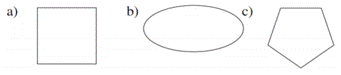Chapter 2.6, Problem 7EElementary Geometry For College St...

7th Edition
Alexander + 2 others
ISBN: 9781337614085

Solutions

Chapter
SectionElementary Geometry For College St...

7th Edition
Alexander + 2 others
ISBN: 9781337614085
Textbook Problem

Which geometric figures have symmetry with respect to a point?To determine

To choose:

The geometric figures having symmetry with respect to a point.

Explanation

Consider the geometric figure (a) and consider point P at the center of the geometric figure.

Figure (1)

Consider arbitrary points A, B, and C on the polygon.

Figure (2)

For each arbitrary point on the polygons, there is second point corresponding to it such that point P is the midpoint of the line formed by the points.

Figure (3)

The points A, B, and C, on the polygon corresponding to points A, B, and C on the such that P is the midpoint of the line from the points.

The geometric figure (a) is symmetric about point P.

Consider the geometric figure (b) and consider point P at the center of the geometric figure.

Figure (4)

Consider arbitrary points A, B, and C on the polygon.

Still sussing out bartleby?

Check out a sample textbook solution.

See a sample solution

The Solution to Your Study Problems

Bartleby provides explanations to thousands of textbook problems written by our experts, many with advanced degrees!

Get Started

Find more solutions based on key concepts